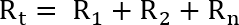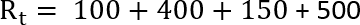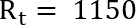Calculating Series Resistance

In this guide, we will be showing you how to calculate the resistance of resistors in series.Resistors are considered to be in a “series” when they connect to each other in a single line.

The amount of current that flows through a series of resistors remains the same. As all resistors connect to one after another, each additional resistor in a series increases the overall resistance.

This is unlike resistors in parallel where current is increased, and the resistance is reduced.

Series Resistance Calculator

Even though calculating the total resistance contributed by resistors in series is a simple process, we have included a handy calculator to make your life even easier.

Total Series Resistance:
Number of resistors:
Resistor 1:
Resistor 2:

To use this series resistance calculator, all you need to do is enter the number of resistors and enter the value of each resistor.

The total resistance provided by your resistors in series will be automatically calculated as you fill in each text box.

Calculating the Total Resistance of Resistors in Series

Here we have included a basic circuit diagram of what resistors in series would typically look like. This diagram should give you a rough idea of what to look out for in a circuit.Unlike resistors in parallel, calculating the total resistance provided by resistors in series is a very straightforward process.

The equation for calculating the resistance of resistors in series is incredibly simple, and is literally, resistor 1 value plus resistor 2 value plus resistor 3 value and so on.Despite its simplicity, we will run you through an example of calculating the total resistance of resistors in series.

Using the Series Resistance Formula

For this section, we will be assuming that you have four resistors in series.

Resistor one has a resistance of 100 ohms, the second a resistance of 400 ohms, another of 150 ohms and the final resistor a resistance of 500 ohms.

As always, the first thing you should do is fill in the equation with all your resistors values.

In this case, all you need to do is write the equation so that each resistors value is added to the next.The next step is to add all the values together. This will give you the total resistance contributed by all your resistors in series.Hopefully, at this point, you will now have an idea on how to quickly calculate the total resistance provided by your resistors in series.

Feel free to drop a comment below if you have any feedback or need any assistance.